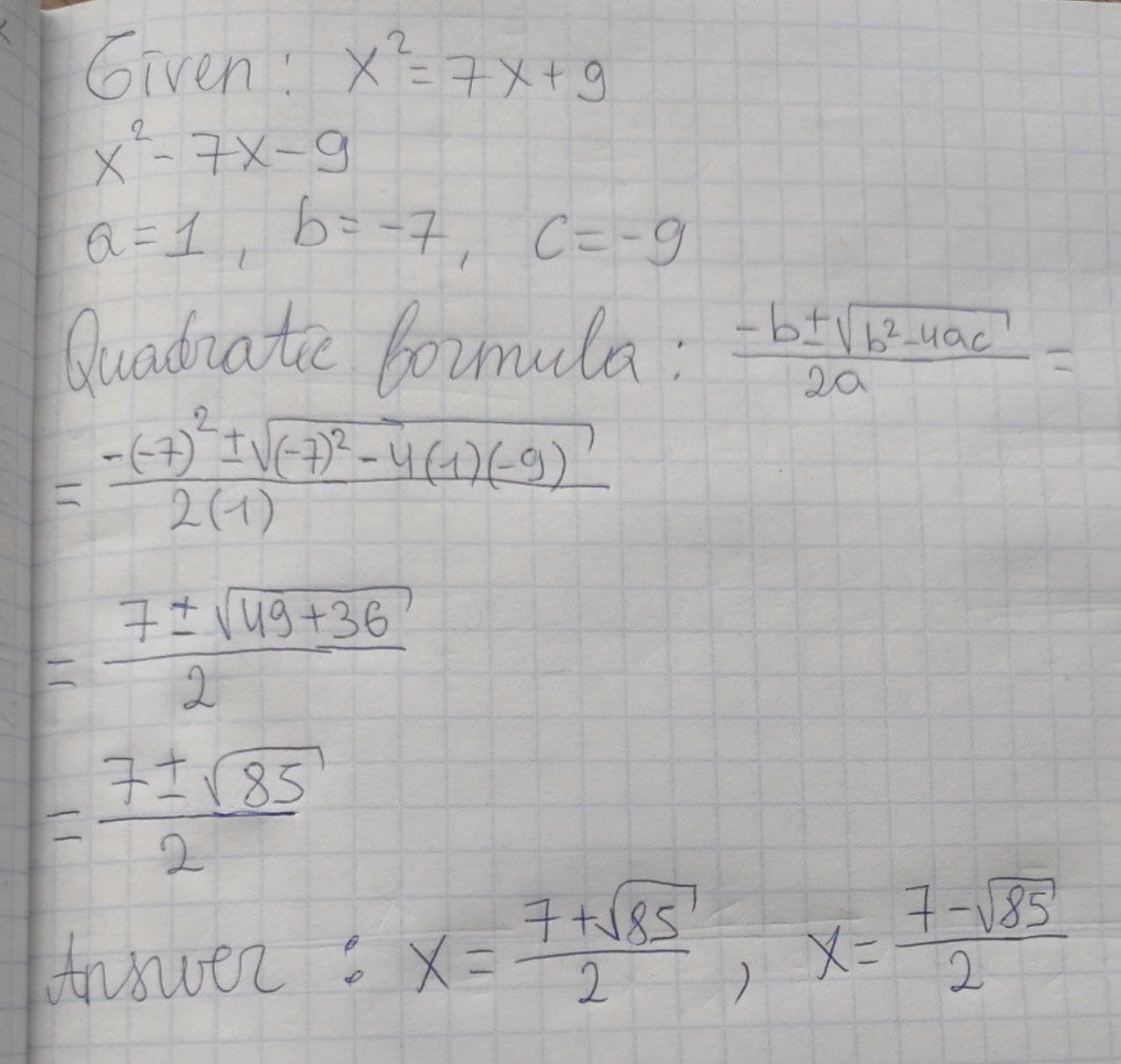# Solve the quadratic by using the quadratic formula x^2 = 7x + 9QuestionSolve the quadratic by using the quadratic formula x^2 = 7x + 92021-02-01
x^2 - 7x - 9 a=1, b=-7, c=-9 Quadratic ormula: x=(-b+-sqrt(b^2-4ac))/(2a) =(-(-7)+-sqrt((-7)^2-4(1)(-9)))/(2(1)) =(7+-sqrt(49+36))/2 =(7+-sqrt85)/2 x=(7+sqrt85)/2, (7-sqrt85)/2### Relevant QuestionsFactoring is used to solve quadratics of the form $$\displaystyle{a}{x}^{{2}}+{b}{x}+{c}={0}$$ when th eroots are rational. Find the roots of the following quadratic functions by factoring:
a) $$\displaystyle{f{{\left({x}\right)}}}={x}^{{2}}-{5}{x}-{14}$$
b) $$\displaystyle{f{{\left({x}\right)}}}={x}^{{2}}-{64}$$
c) $$\displaystyle{f{{\left({x}\right)}}}{6}{x}^{{2}}+{7}{x}-{3}$$Solve quadratic equation using the quadratic formula. $$\displaystyle{x}^{{2}}+{5}{x}+{2}={0}$$Solve quadratic equation using the quadratic formula. $$\displaystyle{3}{x}^{{2}}-{3}{x}-{4}={0}$$Solve the quadratic equation using the quadratic formula. $$\displaystyle{2}{x}^{{2}}-{6}{x}+{5}={0}$$Use the Quadratic Formula to solve $$\displaystyle{8}{x}^{{2}}−{24}{x}+{18}={0}.$$Solve the following quadratic equation $$\displaystyle{6}{x}^{{2}}-{x}-{15}={0}$$$$\displaystyle{4}{\left({x}+{8}\right)}^{{2}}={4}$$The equation $$\displaystyle{m}^{{\frac{{2}}{{3}}}}+{10}{m}^{{\frac{{1}}{{3}}}}+{9}={0}$$ is said to be in __________form, because making the substitution u = __________results in a new equation that is quadratic.Solve the following quadratic equation $$\displaystyle{3}{x}^{{2}}+{5}{x}+{2}={0}$$